Name:    3rd Grade Basic Math Test 3

Multiple Choice
Identify the choice that best completes the statement or answers the question.

1.

Computation – Decimals – RIT 181 –190

26.48
-  15.39
 a. 41.87 d. 11.09 b. 11.11 e. 11.19 c. 11.18

2.

28.45 + 6.27 =
 a. 94.62 d. 35.15 b. 84.72 e. 34.72 c. 9,472

3.

17.32

x     4
 a. 6,928 d. 69.28 b. 4,828 e. 692.8 c. 17.36

4.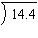a. 7.2 d. 28.8 b. 72 e. 214.4 c. 7

5.

Computation – Fractions – RIT 181 – 190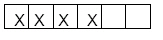Identify the fraction of the bar that has X’s.
 a. 4/2 d. 2/4 b. 4/6 e. 2/6 c. 6/4

6.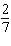-  3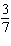a. 3 1/7 d. 3 6/7 b. 9 5/7 e. 2 4/7 c. 2 6/7

7.

Computation – Whole Numbers – RIT 181 – 190

463

- 35
 a. 428 d. 432 b. 438 e. 422 c. 498

8.

4 X 6 = 24
Choose the related division fact.
 a. 246 = 5 d. 12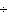6 = 2 b. 246 = 4 e. 424 = 6 c. 624 = 4

9.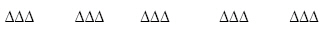Identify the division fact shown in the picture.
 a.d.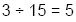b.e.c.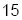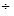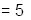10.

2,603
- 1,325
 a. 1,322 d. 1,278 b. 1,288 e. 3.928 c. 1,378

11.

30
x  3
 a. 93 d. 63 b. 60 e. 90 c. 80

12.

205
x  3
 a. 615 d. 651 b. 605 e. 6,015 c. 635

13.

3 x 5 x 4 =
 a. 15 d. 19 b. 60 e. 20 c. 63

14.

Identify the division fact related to 7 X 4 = 28
 a. 287 = 3 d. 357 = 5 b. 428 = 7 e. 728 = 4 c. 287 = 4

15.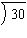a. 6 d. 5 b. 36 e. 24 c. 4

16.

A girl had 21 oranges to put into sacks. She put all of the oranges into 3
sacks. Each sack had the same number of oranges. How many oranges went
into each sack?
 a. 8 d. 18 b. 24 e. 7 c. 6

17.

264
24
156
+ 305
 a. 749 d. 649 b. 739 e. 1,019 c. 744

18.

5,526
- 2,718
 a. 1,818 d. 1,808 b. 2,808 e. 2,212 c. 5,244

19.

5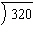a. 60 d. 144 b. 76 e. 514 c. 64

20.

245
1,462

+  210
 a. 1,817 d. 915 b. 817 e. 1,917 c. 1,915

21.a.d.b.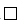e.c.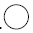22.a.d.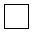b.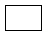e.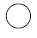c.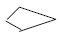23.

Geometry – Shapes and Figures – RIT 151 – 160
Which of these is a triangle?
 a.d.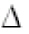b.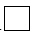e.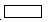c.24.

Geometry – Shapes and Figures – RIT 161 – 170
Which is a cone?
 a.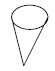d.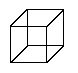b.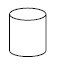e.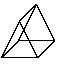c.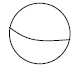25.

Which figure is made of all square sides?
 a.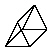c.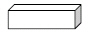b.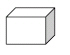26.a. 2 d. 1 b. 4 e. 5 c. 3

27.

Which is a closed figure?
 a.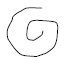d.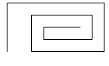b.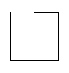e.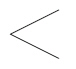c.28.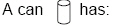a. two circles d. a rectangle side b. square sides e. two square sides c. two triangles